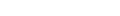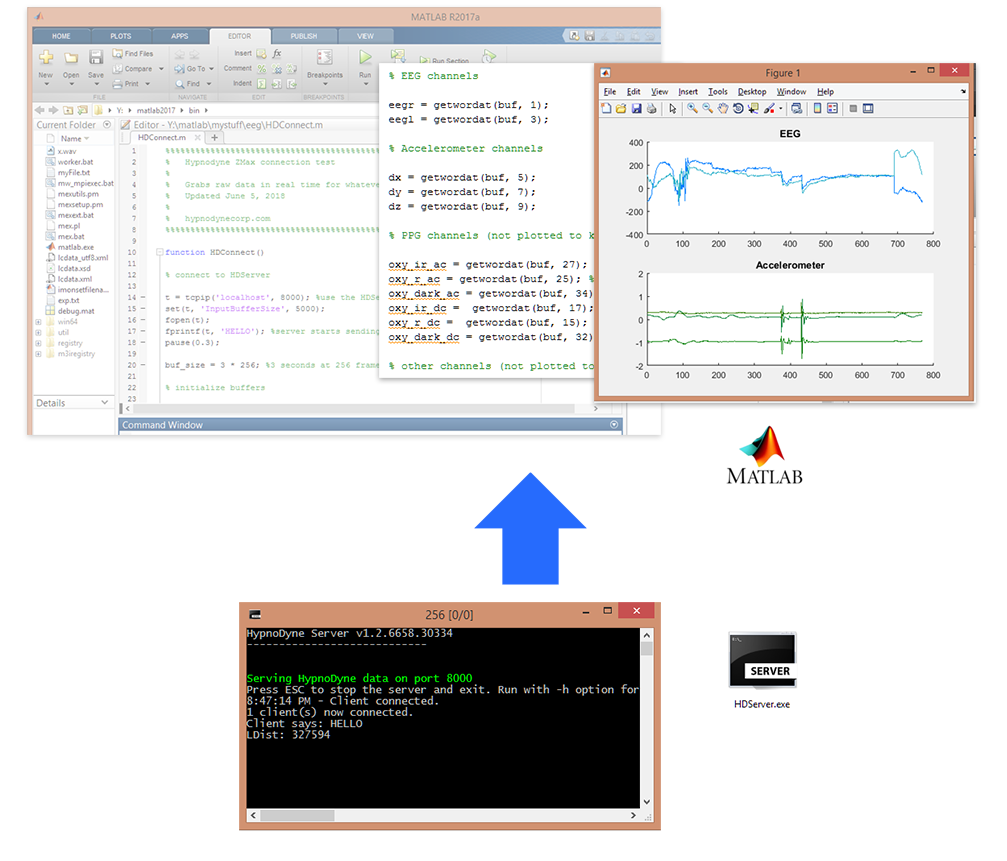## Writing your own softwareZMax exposes a TCP/IP data socket. You can connect to this socket using any programming language (MATLAB, Python, PHP, C++, C#, Java, Visual Basic etc).

All you need to do is open a TCP socket connection to the Hypnodyne Server and parse data bytes.

### MATLAB INTEGRATION

Sample code is provided for MATLAB which clearly demonstrates the meaning of the bytes and how to scale data to obtain decimal values such as microvolts for the EEG channels and so forth.### C# INTEGRATION

Sample functions are included for C# (.NET), please use in conjunction with Microsoft's asynchronous client socket example. A full project will be available soon.

``````

private void AsyncClient_MessageReceived(AsyncClient a, string msg)
{
string[] stringSeparators = new string[] { "\r\n", "\r", "\n", "\n\r" };
string[] lines = msg.Split(stringSeparators, StringSplitOptions.None);
for (int linen = 0; linen < lines.Length; linen++)
{
String line = lines[linen];
bool isDataPacket = false;
if (line != "")
{
if (line.StartsWith("_DONGLE_INSERTED"))
{
if (DongleInserted != null)
DongleInserted(null);
if (Alert != null)
}
if (line.StartsWith("ACKBYTES"))
{
if (AckReceived != null)
{
}
}
if (line.StartsWith("NACKBYTES"))
{
if (NackReceived != null)
{
}
}
if (line.StartsWith("_DONGLE_REMOVED"))
{
if (DongleRemoved != null)
DongleRemoved(null);
if (Alert != null)
}
{
if (ReceiverGone != null)
if (Alert != null)
}
if (line.StartsWith("DEBUG:"))
{

}
else if (line == 'D')
{
String dataline = line.Substring(1);
string[] parts = dataline.Split('.');
byte[] buf = HexToBytes(parts);
int packet_type = buf;
if ((packet_type >= 1) && (packet_type <= 11))
{
if (buf.Length == 40)
{
isDataPacket = true;
ProcessDataPacket(buf, true);
int eegrv = buf * 256 + buf;
int eeglv = buf * 256 + buf;
int dx = buf * 256 + buf;
int dy = buf * 256 + buf;
int dz = buf * 256 + buf;
double scaled_eegr = ScaleEEG(eegrv);
double scaled_eegl = ScaleEEG(eeglv);
double scaled_dx = ScaleAccel(dx);
double scaled_dy = ScaleAccel(dy);
double scaled_dz = ScaleAccel(dz);
}
}
}
}
if (!isDataPacket)
{
if (DebugInfo != null)
DebugInfo(GetDateTime() + " > " + line);
}
}
}

private double ScaleEEG(int x)
{
double y = x;
y = y - 32768;
y = y * (3952);
y = y / 65536;
return y;
}

private double ScaleAccel(int x)
{
double y = x;
y = y * 4 / 4096 - 2;
return y;
}
``````